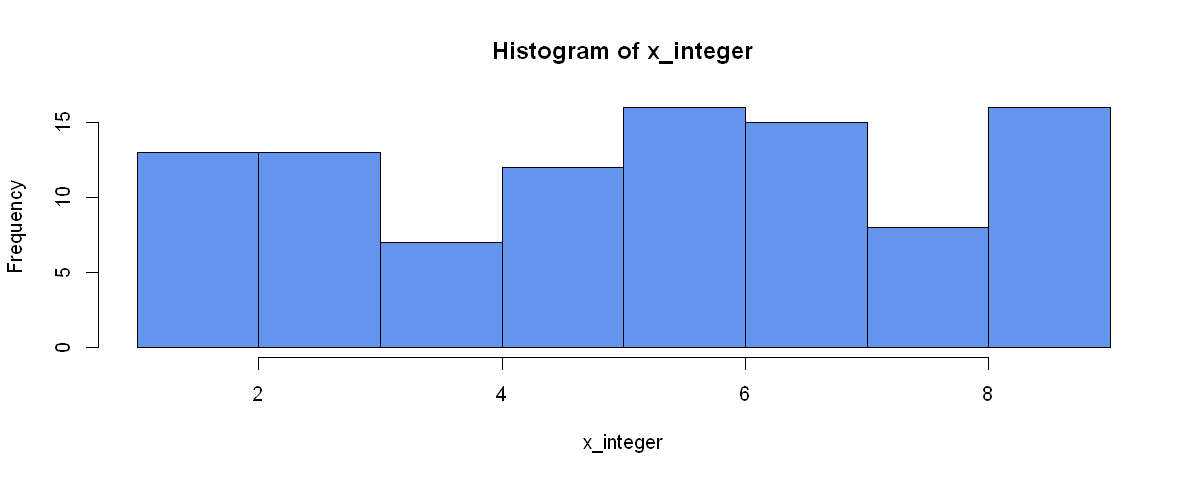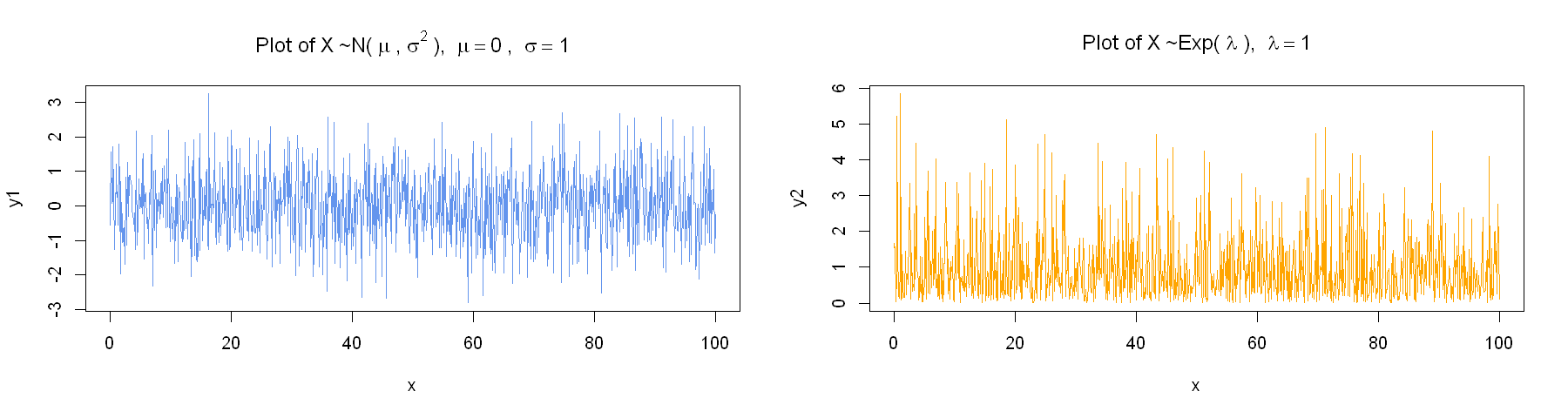# Introduction¶

You can select the box with Code and change it to Markdown in order to write Markdown code. Then press the Run button on the selected cell (or press Ctrl + Enter). You can press Enter to go to a new line in your code block. This will not run your code and allow you to write multiple lines at once.

If you'd like to edit a cell - double click it.

You can have multiple code blocks of Markdown one after the other (note that the use of --- added a horizontal line - we used it as an example to show where the new code block started, you can also see which code belongs to the slected block hi the highlight on the left). This would allow you to section-off some parts of your text, if it gets pretty large.

Since we are using Markdown, we can use similar functionality we use in RStudio's RMarkdown - A quick comparison of Markdown and RMarkdown.

We can still use lists:

• One
• A
• B
• C
• Two
• Three

We can also use Latex for Math notation:

with $:$X_1, X_2, ..., X_3and with $$:$$Y_t = \sum_{j = 1}^t \epsilon_t^2$$As well as matrices:$$ \begin{bmatrix} \alpha& \beta^{*}\\ \gamma^{*}& \delta \end{bmatrix}, \quad \begin{pmatrix} \alpha& \beta^{*}\\ \gamma^{*}& \delta \end{pmatrix} Aligned equations: \begin{align} Y_{1,t} &= \alpha_1 + \beta_1 X_{1,t} + \epsilon_{1,t} \\ Y_{2,t} &= \alpha_1 + \beta_1 X_{1,t} + \epsilon_{1,t} \end{align} and \begin{align} Population_t &= \alpha_1 + \gamma_1 X_{1,t} + \gamma_2 X_{1,t-1} \\ Price_t &= \alpha_2 + \beta_1 Z_{1,t} \end{align} equation systems: f(n) = \begin{cases} n/2 &\mbox{if } n \equiv 0 \\ (3n +1)/2 & \mbox{if } n \equiv 1. \end{cases} \pmod{2} $$Regression models:$$ \text{wage} = \beta_0 + \beta_1 \cdot \text{educ}^2 + \epsilon$estimated regressions, along with their standard errors:$ \underset{(se)}{\widehat{\log(\text{wage})}} = \underset{(0.0702)}{1.5968} + \underset{(0.0048)}{0.0988} \cdot \text{educ} \$

(sometimes the formulas might not generate at first run - double click the code cell and select Run to again evaluate the code)

Another difference from RMarkdown in Rstudio is that we do not need to re-generate the whole document each time!

# Python code¶

As mentioned, we can run R code here as well by inserting a new code block with Code instead of Markdown selected.

In :
set.seed(123)
#
nsample = 1000
eps <- rnorm(n = nsample)
print(mean(eps))

 0.01612787


Note that we can re-run this code chunk to get the same average value. If we try to run the next block more than once, we will get different results, much like with Rmarkdon in R:

In :
eps <- rnorm(n = nsample)
print(mean(eps))

 0.04246525


Note that, if we run the 1st block and then the 2nd block - we will get the same results as long as they are run in the same order only once.

As with Python, always set a seed before random number generation in the same block, in order to make sure that your results are reproducible!

We can also define our functions in one code block:

In :
my_add <- function(x, y){
x = x + 1
y = y + 1
return(x + y)
}


And use them in a different code block:

In :
print(my_add(1, 2))

 5


We can also plot our data. We can see the value in the output below.

# Plot of a histogram¶

In :
set.seed(123)
#
x_integer <- sample(1:9, 100, replace = T)

In :
print(x_integer)

   3 3 2 6 5 4 6 9 5 3 9 9 9 3 8 7 9 3 4 1 7 5 7 9 9 7 5 7 5 6 9 2 5 8 2 1 9
 9 6 5 9 4 6 8 6 6 7 1 6 2 1 2 4 5 6 3 9 4 6 9 9 7 3 8 9 3 7 3 7 6 5 5 8 3
 2 2 6 4 1 6 3 8 3 8 1 7 7 7 6 7 5 6 8 5 7 4 3 9 7 6


After simulating some data, we can plot its histogram. A very basic example is below (see the lectures for more customizable plot examples):

In :
# We need to set the default output plot sizes in this notebook:
options(repr.plot.width = 10)
options(repr.plot.height = 4)


Note: This the way to change the plot output size when using R in JupyterLab. For Python - the figure size can be changed in plt.figure().

In :
hist(x_integer, col = "cornflowerblue")As we can see from the above plot - the data histogram is ploted using some default color and style parameters.

After the plot we could add some comments on what we see in this plot, whether it looks like from a normal distrbution and so on.

# Multiple plots example¶

In :
set.seed(123)
#
x  <- seq(from = 0, to = 100, length.out = nsample)
y1 <- rnorm(n = nsample)
y2 <- rexp(n = nsample)

In :
print(mean(y1))
print(mean(y2))

 0.01612787
 0.9836951


We can see from the output that the default value of the mean is:

• around 0 for the rnorm() function;
• around 1 for the rexp() function.
In :
# If we want a different-sized plot - we need to change the options
options(repr.plot.width = 15)
options(repr.plot.height = 4)
# Then, form this point on - all of the plots will be in this new size:

In :
#a 1-row, 2-column figure:
par(mfrow=c(1, 2))
# plots are added in the order that we plot them:
plot(x, y1, col = "cornflowerblue", type = "l",
main = bquote("Plot of"~X~"~N("~mu~","~sigma^2~"), "~mu==0~", "~sigma==1))
plot(x, y2, col = "orange", type = "l",
main = bquote("Plot of"~X~"~Exp("~lambda~"), "~lambda==1))Again, examine the lecture notes/lecture slides for additional ways to plot the data.

In [ ]: